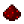# bit.brshiftFunction bit.brshift Shifts a number right arithmetically by a specified number of bits Syntax bit.brshift(number n, number bits) Returns number the value of n shifted right by bits bits, with the shifted-in bits being equal to the original number’s 31st bit, which is equivalent to ⌊n÷2bits⌋ Part of ComputerCraft API bitExample Shift the number 73 (1001001) right by 2 bits, yielding 18 (10010) Code ```print(bit.brshift(73, 2)) ``` Output 18Example Shift the number 2,147,483,648 (10000000000000000000000000000000) right by 2 bits, yielding 3,758,096,384 (11100000000000000000000000000000) Code ```print(bit.brshift(2147483648, 2)) ``` Output 3758096384